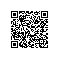# 笔试题目“翻转字符串”的实现

## 笔试题目

//写一个函数，将字符串翻转，翻转方式如下：“I am a student”反转成“student a am I”，不借助任何库函数。

## 算法

 1 #include <stdio.h>
2
3 void main()
4 {
5     char str[]="I am a student"; 7     printf(str);
8     printf("\n");
9
10     char *p,*q;
11     char temp;
12     p=q=str;14     while(*q!='\0')
15     {
16         q++;
17     }
18     q--;
19     while(p<=q)
20     {
21         temp=*p;
22         *p=*q;
23         *q=temp;
24         p++;
25         q--;
26     }//反转整个字符串
27
28     printf(str);
29     printf("\n");
30
31     q=str;//指针指向开始位置
32     char *s,*t;
33     s=t=str;
34     while(*q!='\0')
35     {
36         if(*q==' ')
37         {
38             t--;
39             while(s<=t)
40             {
41                 temp=*t;
42                 *t=*s;
43                 *s=temp;
44                 s++;
45                 t--;
46             }//反转局部字符串
47
48             s=q+1;
49             t=q;
50         }52         q++;
53         t++;
54     }
55
56     printf(str);
57     printf("\n");
58 }

## 改进if(*q==' ')

if(*q==' '||*(q+1)=='\0')
{
t--;
if(*(q+1)=='\0')//处理最后一个字串
t++;## 代码已处理最后字串的完整代码《程序员面试宝典》实现方法使用钉钉扫一扫加入圈子
+ 订阅